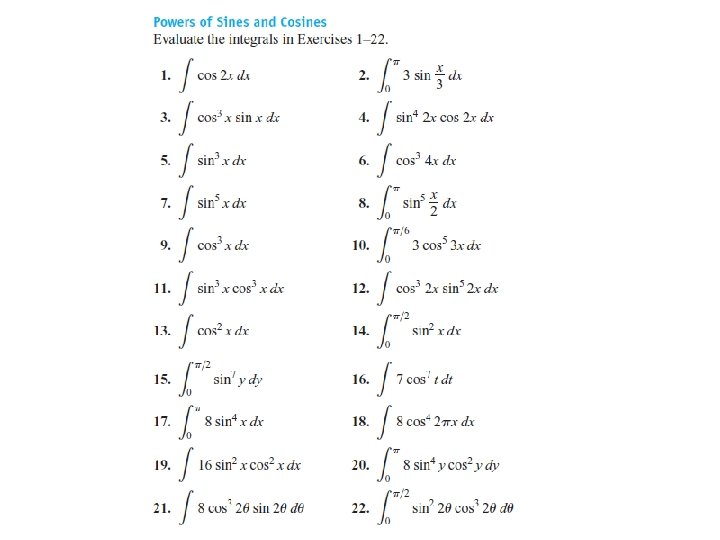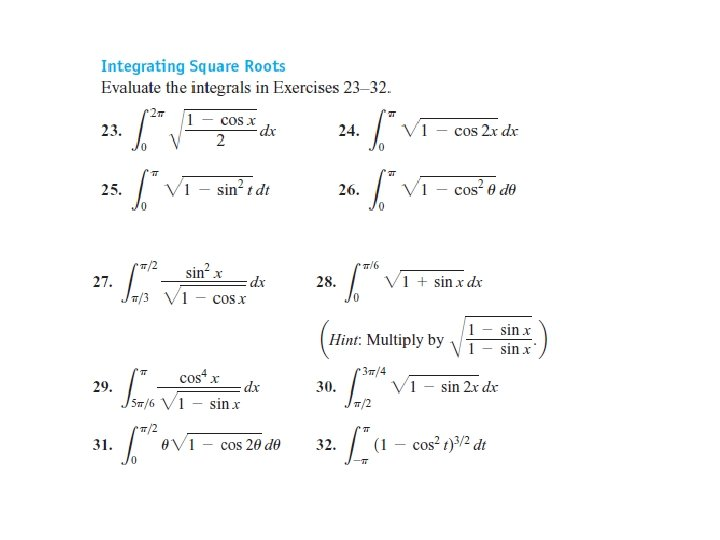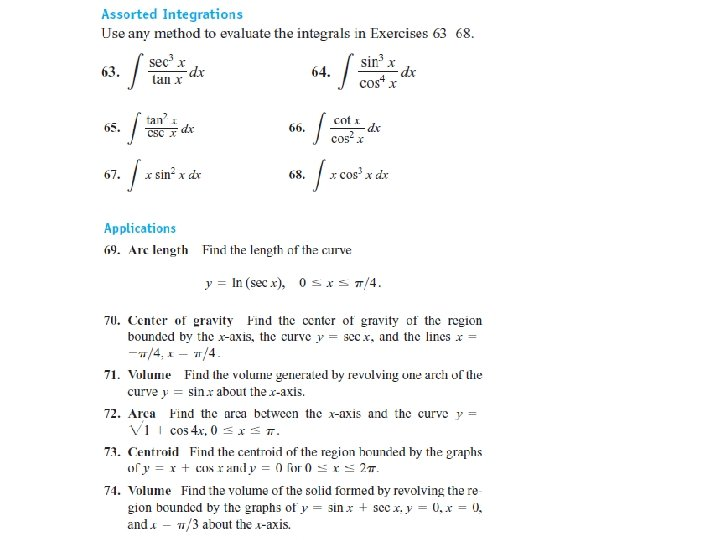# SEC 8 2 TRIGONOMETRIC INTEGRALS Example Find TRIGONOMETRIC

• Slides: 26SEC 8. 2: TRIGONOMETRIC INTEGRALS Example FindTRIGONOMETRIC INTEGRALS 1 1 2 2 to express the remaining factors in terms of sin to express the remaining factors in terms of cos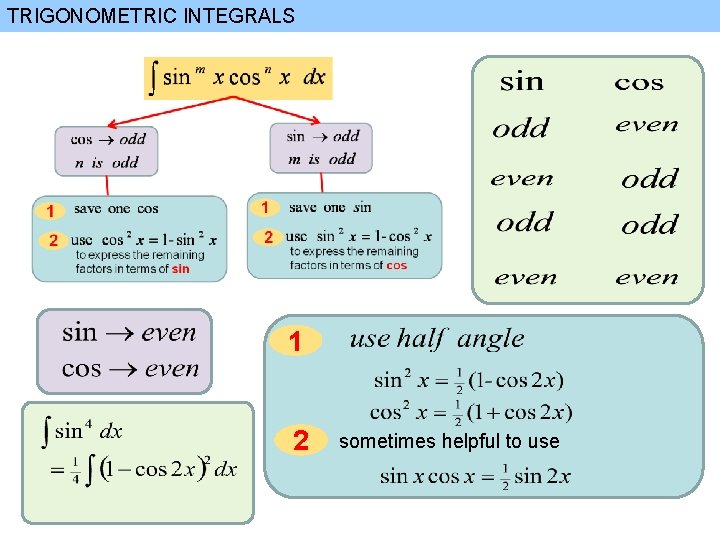TRIGONOMETRIC INTEGRALS 1 2 sometimes helpful to use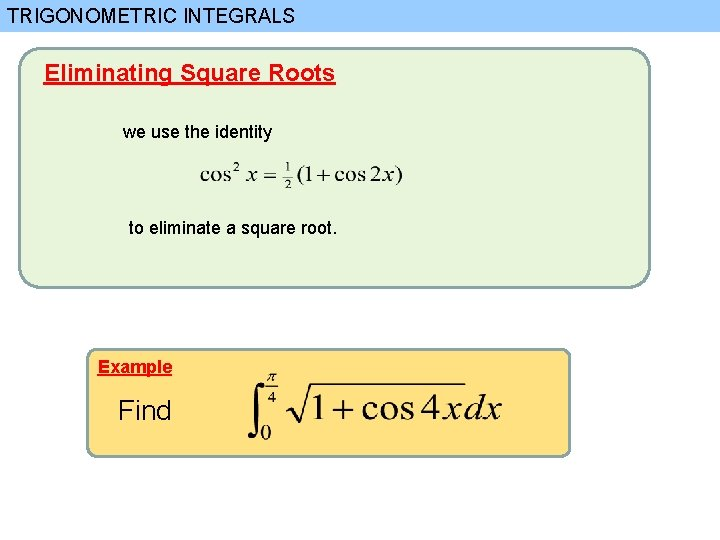TRIGONOMETRIC INTEGRALS Eliminating Square Roots we use the identity to eliminate a square root. Example Find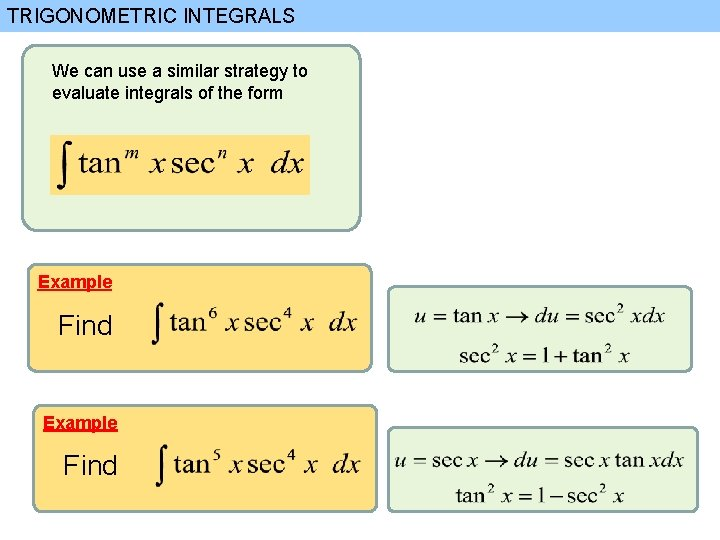TRIGONOMETRIC INTEGRALS We can use a similar strategy to evaluate integrals of the form Example Find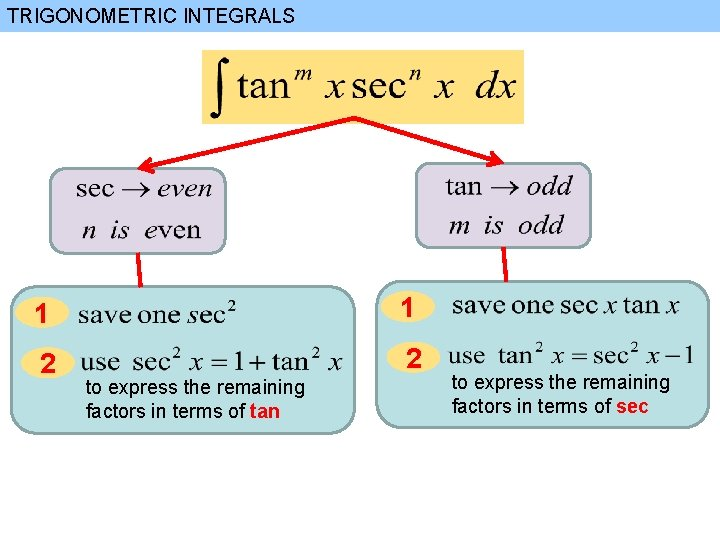TRIGONOMETRIC INTEGRALS 1 1 2 2 to express the remaining factors in terms of tan to express the remaining factors in terms of sec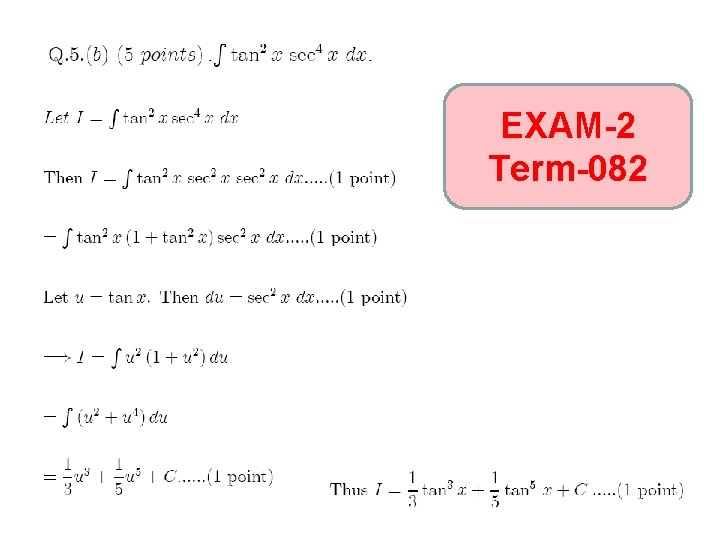EXAM-2 Term-082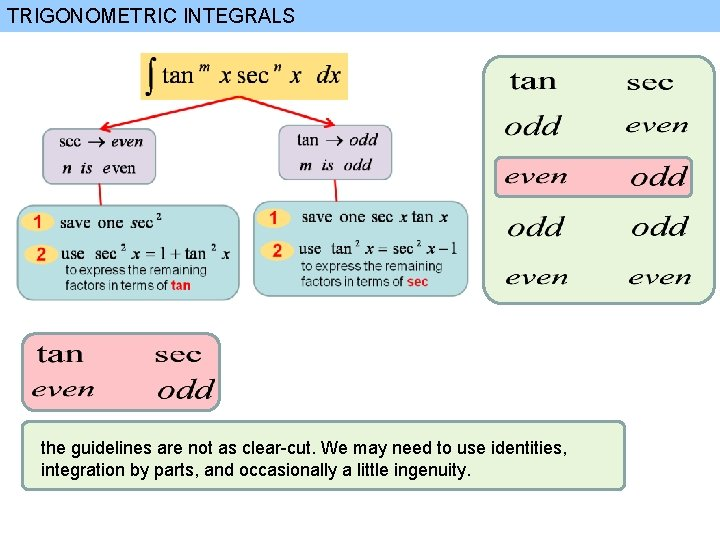TRIGONOMETRIC INTEGRALS the guidelines are not as clear-cut. We may need to use identities, integration by parts, and occasionally a little ingenuity.TRIGONOMETRIC INTEGRALS the guidelines are not as clear-cut. We may need to use identities, integration by parts, and occasionally a little ingenuity. Example Find If an even power of tangent appears with an odd power of secant, it is helpful to express the integrand completely in terms of sec x Powers of sec x may require integration by parts, as shown in the following example.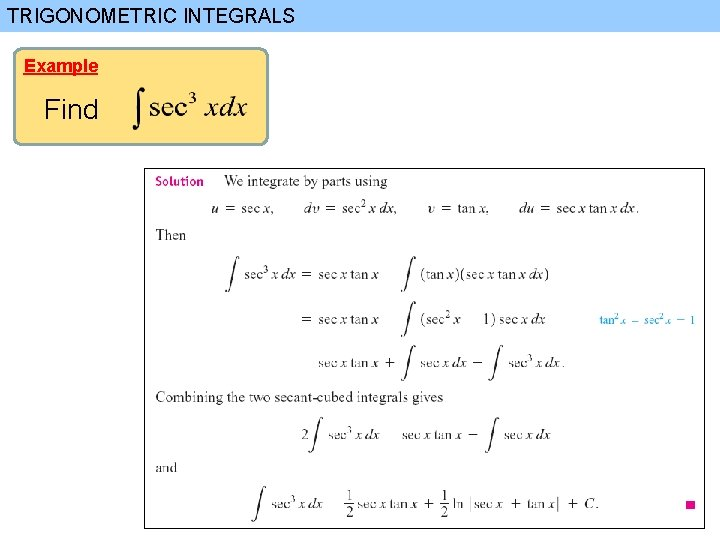TRIGONOMETRIC INTEGRALS Example Find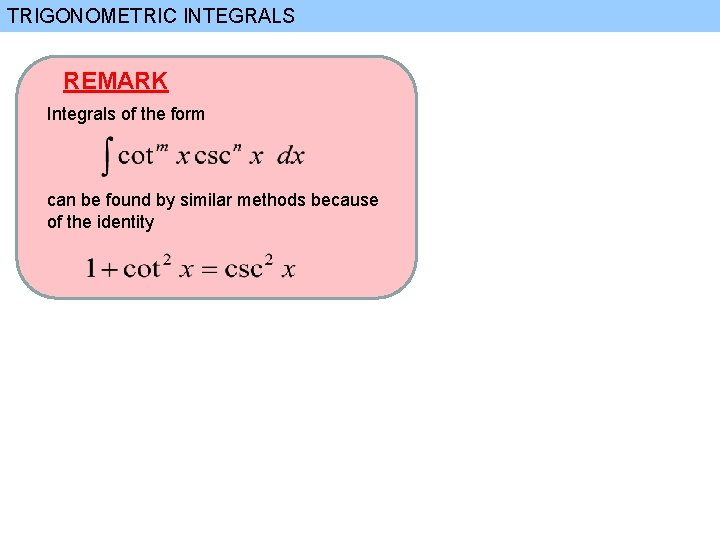TRIGONOMETRIC INTEGRALS REMARK Integrals of the form can be found by similar methods because of the identity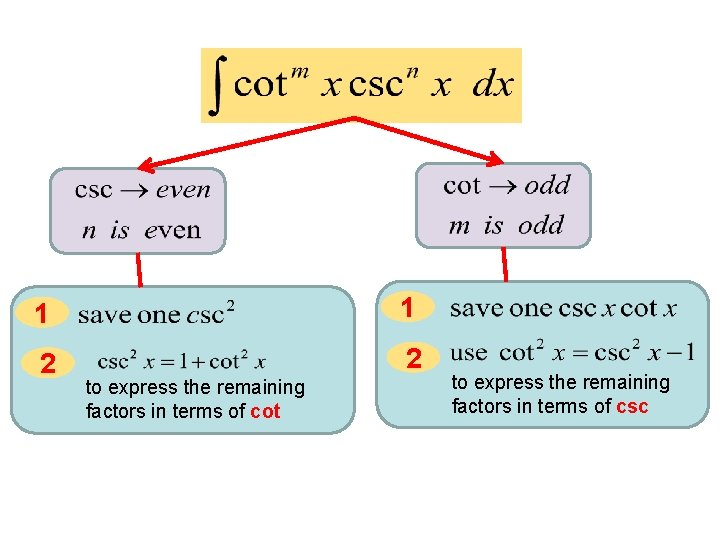1 1 2 2 to express the remaining factors in terms of cot to express the remaining factors in terms of csc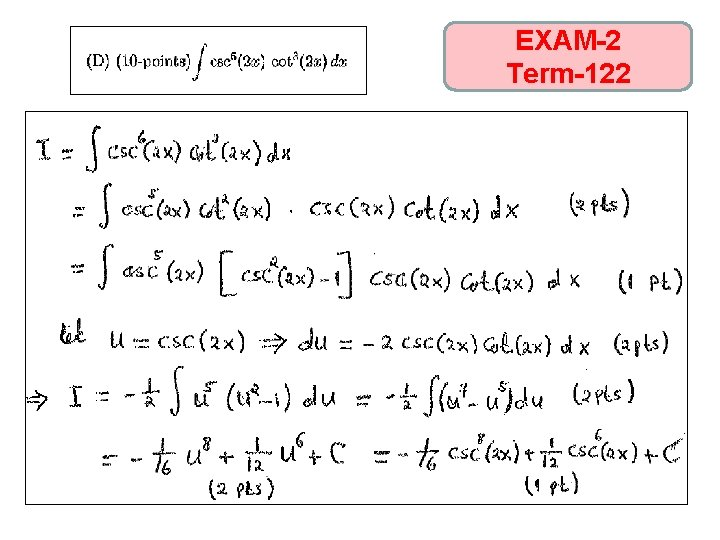EXAM-2 Term-122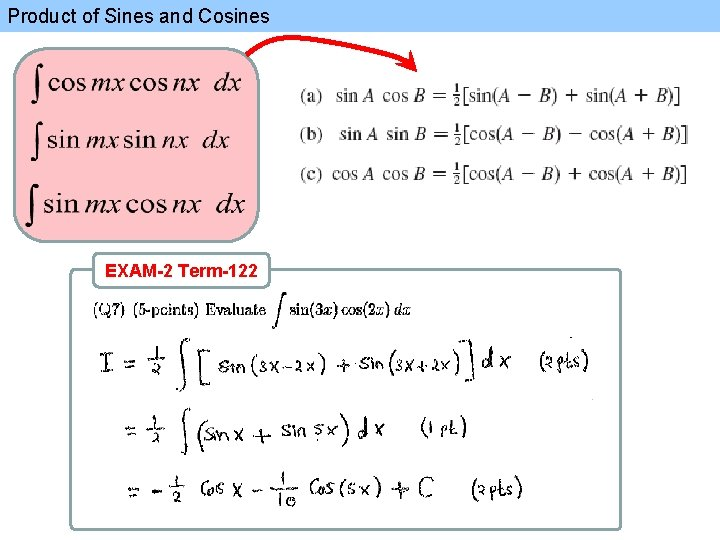Product of Sines and Cosines EXAM-2 Term-122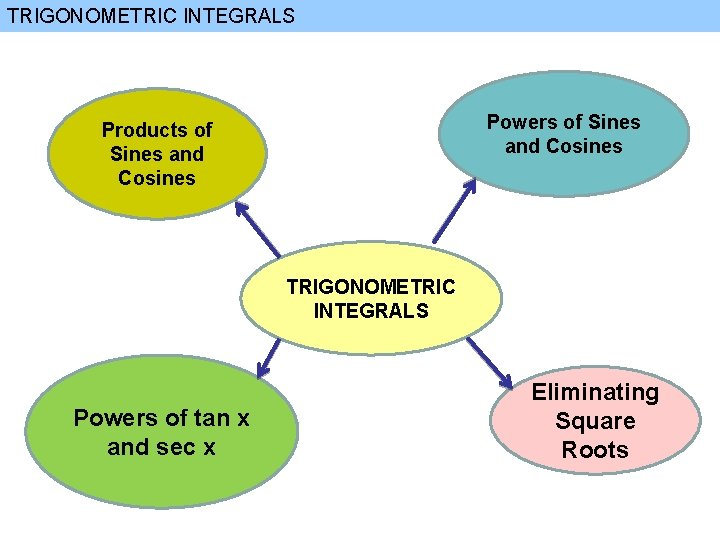TRIGONOMETRIC INTEGRALS Powers of Sines and Cosines Products of Sines and Cosines TRIGONOMETRIC INTEGRALS Powers of tan x and sec x Eliminating Square RootsEXAM-2 Term-092TRIGONOMETRIC INTEGRALS function of tan and sec function of Sines and Cosines TRIGONOMETRIC INTEGRALS function of cot and csc EXAM-2 Term-092EXAM-2 Term-092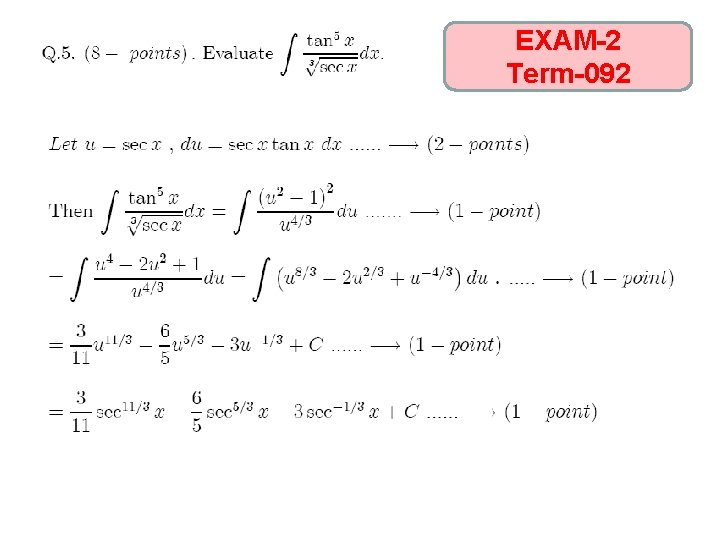EXAM-2 Term-092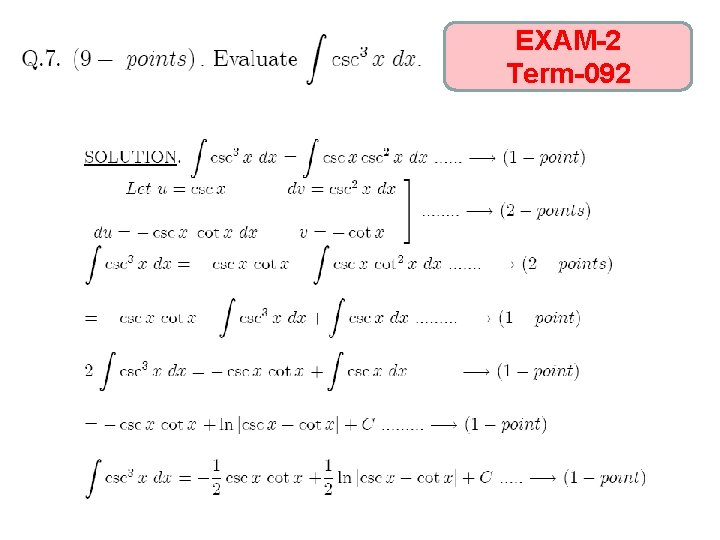EXAM-2 Term-092